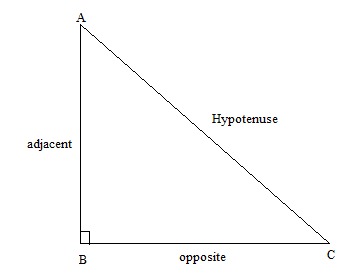QuestionAnswers

# The term trigonometry was first invented by the German mathematician:A. William Rowan HamiltonB. EuclidC. NewtonD. Bartholomaeus pitiscus.Verified
129k+ views
Hint: In this question, we will see the basics of trigonometry like what is trigonometry, its history and its some of the basic functions. In the history of trigonometry, we will come to know about the mathematician who coined the word “trigonometry”.

Let us first see what trigonometry is.
Trigonometry is made up of two greek words “trigonon” which means “triangle” and “metron” which means “measure”. It is the branch of mathematics which deals with the relationship between side lengths and angles of the triangle.
In trigonometry we basically learn about the trigonometric ratios.Sine function(sin)- it is given as the ratio of side opposite the angle and hypotenuse.
sinA = $\dfrac{{BC}}{{AC}}$

Cosine function (cos), defined as the ratio of the adjacent leg (the side of the triangle joining the angle to the right angle) to the hypotenuse.
cosA = $\dfrac{{AB}}{{AC}}$
Tangent function (tan), defined as the ratio of the opposite leg to the adjacent leg.
tanA = $\dfrac{{BC}}{{AB}}$

The term trigonometry was first coined by the German trigonometry, astronomer and theologian Bartholomaeus Pitiscus.
Pitiscus edited the “Thesaurus mathematicus”(1613) in which he improved the trigonometric tables of “George Joachim Rheticus”.
So,Option D is the correct answer.

Note:
The hypotenuse is the side opposite to the 90 degree angle in a right triangle and is the largest side of the triangle. The reciprocal of sine, cosine and tangent are cosecant(csc), secant(sec) and cotangent(cot) respectively.
$\csc A = \dfrac{1}{{\sin A}} = \dfrac{{AC}}{{BC}}$
$\sec A = \dfrac{1}{{\cos A}} = \dfrac{{AC}}{{AB}}$
$\cot A = \dfrac{1}{{\tan A}} = \dfrac{{AB}}{{BC}}$